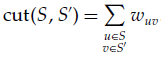# MaxCut Problem

Martí, Duarte and Laguna (2009)
University of Valencia (Spain), University Rey Juan Carlos (Spain) and University of Colorado at Boulder (USA)

# Problem Description

Consider a graph G = (V, E) with vertex set V = [1,...,n] and edge set E. Let wij be the weight associated with edge (i, j) in E. A cut(S, S') is a partition of V into two sets S, S' = V \ S, and its value cut(S, S') is given by the expression:The max-cut problem consists of finding a cut in G with maximum value. Karp (1972) showed that the max-cut is an NP-hard problem.

# State of the Art Methods

The most relevant metaheuristics developed to solve this problem are:

• SS: Scatter Search. Martí et al (2009)
• CirCut: Heuristic based on problem relaxations. Burer et al (2001)
• VNSPR: Variable Neighbourhood Search and Path Relinking. Festa et al (2002)

# MaxCut Instances

We have compiled a comprehensive set of benchmark problems including all problem instances which have so far been used for conducting computational experiments for the MaxCut problem. We give a brief description of the origin and the characteristics of the groups of problems.

• Set 1:This set consists of instances generated by Helmberg and Rendl (2000) who used rudy, a machine-independent graph generator by Giovanni Rinaldi, to create 54 instances ranging from n = 800 to 3,000. They consist of toroidal, planar, and random graphs with weights taking the values 1, 0, or −1. Burer et al. (2001) and Festa et al. (2002) used these graphs in their experiments. Download.
• Set2: This set contains 30 instances described in Festa et al. (2002). The first 10 are small-size instances with an average of 128 vertices and 300 edges, the second 10 instances are medium-size graphs with 1,000 vertices and density equal to 0.60%, and the last 10 are large-size instances with 2,744 vertices and density equal to 0.22%. The weight values are either 1, 0, or −1. Download.
• Set 3: The four instances in this set come from the 7th DIMACS Implementation Challenge. The number of vertices ranges from 512 to 3,375 and the number of edges from 1,536 to 10,125. Burer et al. (2001) used all four instances in their experiments. Festa et al. (2002) considered only one. Download.

# Computational Experience

We have performed an intensive experimentation with the best know methods to compute the best known values for the instances. We have also reviewed related literature searching for better values than these. The final best known values for the instances can be downloaded here.

We performed a computational comparison of the state of the art methods on the instances. The experiments were performed in a Dual Intel Xeon at 3.06 GHz with 3.2 GiB of RAM. It can be downloaded in Excel.

# References

• Burer, S., R. D. C. Monteiro, Y. Zang. 2001. Rank-two relaxation heuristics for max-cut and other binary quadratic programs. SIAM J. Optim. 12 503–521
• Festa, P., P. M. Pardalos, M. G. C. Resende, C. C. Ribeiro. 2002. Randomized heuristics for the max-cut problem. Optim. Methods Software 7 1033–1058
• Helmberg, C., F. Rendl. 2000. A spectral bundle method for semidefinite programming. SIAM J. Optim. 10 673–696
• Karp, R. M. 1972. Reducibility among combinatorial problems. R. Miller, J. Tatchers, eds. Complexity of Computer Computations. Plenum Press, New York, 85–103
• Martí, R., A. Duarte, M. Laguna. 2009. Advanced Scatter Search for the Max-Cut Problem. INFORMS Journal on Computing 21(1), pp. 26–38